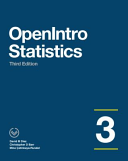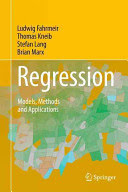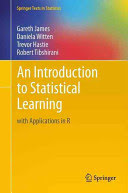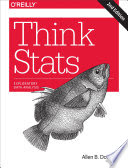### Statistical Data Analysis

#### Course Description

Statistics plays a vital role in every human activity, while the ability to use statistical inferential methods and interpret the results are essential in engineering and science. This course gives a comprehensive introduction to the methods and practices of computer-based statistical data analysis. The course covers and intertwines four integral aspects of statistical analysis: data, statistical methods, mathematical foundations, and interpretation of results. The first part of the course gives an overview of statistical methods, approaches to data description, and data visualization and exploration methods. The second part is devoted to the foundations of statistical inference and covers the selection, application, and adequacy of parametric statistical tests for numeric and categorical data. The third part considers more advanced topics, such as non-parametric statistics, analysis of variance, and correlation analysis. All concepts are illustrated with examples and problem sets on real data in programming languages R and Python.

#### Learning Outcomes

1. Define main notions in the statistical data analysis
2. Explain mathematical backgrounds of main statistical procedures
3. Apply procedure of data preparation and visualization
4. Apply statistical test on real data
5. Analyze the relation between statistical variables by applying regression analysis and correltion analyis
6. Justify the adequacy of statistical inference for given data
7. Interpret the results of statistical data analysis and explain their practical meaning

#### Forms of Teaching

Lectures

3 hours per week

Exams

2 exams

Laboratory Work

1 hour per week, focused into 4 sessions per 3 hours each

Consultations

Assisting the students in completing their projects.

Continuous Assessment Exam
Seminar/Project 50 % 40 % 0 % 40 %
Mid Term Exam: Written 50 % 30 % 0 %
Final Exam: Written 50 % 30 %
Exam: Written 50 % 60 %
##### Comment:

The 50% threshold on the midterm and final exam scores is applied to the sum of these two scores.

#### Week by Week Schedule

1. Introduction and motivation (the role of statistics in science and practice; taxonomy of statistical methods; overview of the literature and tools)
2. Data (measurement scales; describing numerical and categorical data; outliers; data transformation)
3. Data visualization and exploration (scatter plot, histograms, q-q plot)
4. Introduction to statistical inference (population, sample, and sampling; observational and experimental study designs)
5. Principles of statistical inference (hypoteses; tests; pvalue; significance)
6. Statistical inference for numerical data (comparing the means; paired data; tests for the variance)
7. Statistical inference for categorical data (testing proportions; contingency tables; chi-square test)
8. Choosing the right test (sample size; test conditions and limitations)
9. Introduction to nonparametric statistics (methods overview; sign test; Mann-WhitneyWilcoxon test; Wilcoxon signed-rank test; pros and cons)
10. Resampling methods (permutation test; principles of boostrapping)
11. Introduction to analysis of variance (single factor ANOVA, ANOVA table, F-test, DurbinWatson test; Barlett's test; Bonferroni correction)
12. Regression and correlation analysis (statistical inference using regression; residual analysis; confidence intervals)
13. Regression and correlation analysis (multiple regression; nonlinear regression; logistic regression)
14. Alternative approaches to data analysis (bayesian vs. frequentist statistics; examples of bayesian modeling and inference)
15. Wrap-up and recommendations for further studies

#### Study Programmes

Computer Science (module)
Elective Courses (6. semester)
Electronic and Computer Engineering (module)
Elective Courses (6. semester)
Information Processing (module)
Elective Courses (6. semester)
Software Engineering and Information Systems (module)
Elective Courses (6. semester)
Telecommunication and Informatics (module)
Elective Courses (6. semester)

#### LiteratureŽeljko Pauše (1992.), Uvod u matematičku statistiku, Školska knjigaDavid M. Diez, Crhisopher D. Barr, Mine CerinkayaRundel (2015.), OpenIntro Statistics, OpenIntro
Mirta Benšić, Nenad Šuvak (2013.), Primijenjena statistika, Sveučilište J. J. Strossma yeraL. Fahrmeir, T. Kneib, S. Lang, B. Marx (2013.), Regression: Models, Methods and Applications, SpringerG. James, Daniela Witten,Tre vor Hastie, Robert Tibshirani (2013.), An Introduction to Statistical Learning with Applications in R, SpringerAllen B. Downey (2014.), Think Stats, "O'Reilly Media, Inc."

#### General

ID 155246
Summer semester
4 ECTS
L0 English Level
L1 e-Learning
45 Lectures
15 Exercises
0 Laboratory exercises
0 Project laboratory